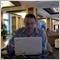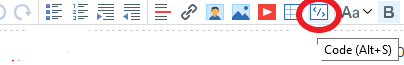# Errors with Metatrader 5 build 208514

EA code was working for the past 2 weeks, after doing a metatrader 5 update I  updated a variable and compiled which generated a few errors. All errors stated "function already defined and has different type."

Here is an example of one of the class definition:

void ChangeTrendline(Channel &ch);

Here is an example of function with body which is giving the error:

Trendline::ChangeTrendline(int index)

{

}

If I add the body to the definition then the error goes away.

example:

void ChangeTrendline(int index){myindex = index;}

But this is not convenient when the function body has multiple lines of code.

Any help would be really appreciated.38509

In the definition you have a parameter "Channel &ch" and in the implementation you have a parameter "int index". Obviously that doesn't match.14

Alain Verleyen:
In the definition you have a parameter "Channel &ch" and in the implementation you have a parameter "int index". Obviously that doesn't match.A

Sorry about that I copied the code incorrectly. Was trying to simplify my example and left that in there.

Here is an example of one of the class definition:

void ChangeTrendline(int index);

Here is an example of function with body which is giving the error:

Trendline::ChangeTrendline(int index)

{

}

If I add the body to the definition then the error goes away.

example:

void ChangeTrendline(int index){myindex = index;}

But this is not convenient when the function body has multiple lines of code.

Any help would be really appreciated.14

kdouglas:

Sorry about that I copied the code incorrectly. Was trying to simplify my example and left that in there.

Here is an example of one of the class definition:

void ChangeTrendline(int index);

Here is an example of function with body which is giving the error:

Trendline::ChangeTrendline(int index)

{

}

If I add the body to the definition then the error goes away.

example:

void ChangeTrendline(int index){myindex = index;}

But this is not convenient when the function body has multiple lines of code.

Any help would be really appreciated.

Figured it out!

Build 2085 now requires you to add the type to the function for void.

Before Build 2085 you could do this.

void ChangeTrendline(int index);

Trendline::ChangeTrendline(int index)

{

}

For build 2085

definition:

void ChangeTrendline(int index);

function body:

void  Trendline::ChangeTrendline(int index)

{

}Moderator
111252